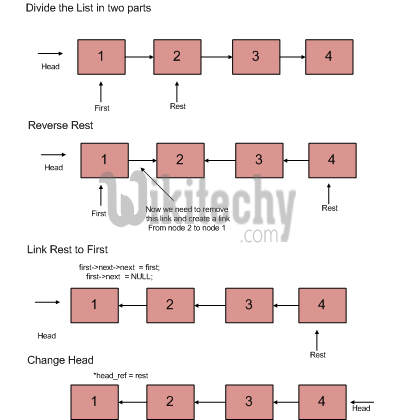# C Algorithm – Write a function to reverse a linked list

C Algorithm - Write a function to reverse a linked list - Linked List - Given pointer to the head node of a linked list, the task is to reverse

Examples:

```Input : Head of following linked list
1->2->3->4->NULL
Output : Linked list should be changed to,
4->3->2->1->NULL

1->2->3->4->5->NULL
Output : Linked list should be changed to,
5->4->3->2->1->NULL

Input : NULL
Output : NULL

Input  : 1->NULL
Output : 1->NULL```

Iterative Method
Iterate trough the linked list. In loop, change next to prev, prev to current and current to next

C Programming:

``````#include<stdio.h>
#include<stdlib.h>

struct node
{
int data;
struct node* next;
};

/* Function to reverse the linked list */
{
struct node* prev   = NULL;
struct node* next;
while (current != NULL)
{
next  = current->next;
current->next = prev;
prev = current;
current = next;
}
}

/* Function to push a node */
void push(struct node** head_ref, int new_data)
{
/* allocate node */
struct node* new_node =
(struct node*) malloc(sizeof(struct node));

/* put in the data  */
new_node->data  = new_data;

/* link the old list off the new node */

/* move the head to point to the new node */
}

/* Function to print linked list */
{
while(temp != NULL)
{
printf("%d  ", temp->data);
temp = temp->next;
}
}

/* Driver program to test above function*/
int main()
{

getchar();
}``````
```Given linked list
85 15 4 20
20 4 15 85```

Time Complexity: O(n)
Space Complexity: O(1)

Recursive Method:

```   1) Divide the list in two parts - first node and rest of the linked list.
2) Call reverse for the rest of the linked list.C Programming:

``````void recursiveReverse(struct node** head_ref)
{
struct node* first;
struct node* rest;

/* empty list */
return;

/* suppose first = {1, 2, 3}, rest = {2, 3} */
rest  = first->next;

/* List has only one node */
if (rest == NULL)
return;

/* reverse the rest list and put the first element at the end */
recursiveReverse(&rest);
first->next->next  = first;

/* tricky step -- see the diagram */
first->next  = NULL;

/* fix the head pointer */
}``````

Time Complexity: O(n)
Space Complexity: O(1)

C++ Programming:

``````// A simple and tail recursive C++ program to reverse
#include<bits/stdc++.h>
using namespace std;

struct node
{
int data;
struct node *next;
};

void reverseUtil(node *curr, node *prev, node **head);

// This function mainly calls reverseUtil()
// with prev as NULL
{
return;
}

// A simple and tail recursive function to reverse
// a linked list.  prev is passed as NULL initially.
void reverseUtil(node *curr, node *prev, node **head)
{
/* If last node mark it head*/
if (!curr->next)
{

/* Update next to prev node */
curr->next = prev;
return;
}

/* Save curr->next node for recursive call */
node *next = curr->next;

/* and update next ..*/
curr->next = prev;

}

// A utility function to create a new node
node *newNode(int key)
{
node *temp = new node;
temp->data = key;
temp->next = NULL;
return temp;
}

// A utility function to print a linked list
{
{
cout << head->data << " ";
}
cout << endl;
}

// Driver program to test above functions
int main()
{
return 0;
}``````

Output:

```Given linked list
1 2 3 4 5 6 7 8